# Ferris wheel

Ferris wheel reaches to 22 m tall and moves at the speed of 0.5m/s. During one drive wheel rotates three times. What is the total drive time?

Result

t =  414.7 s

#### Solution:Leave us a comment of example and its solution (i.e. if it is still somewhat unclear...):

Showing 0 comments:Be the first to comment!#### To solve this example are needed these knowledge from mathematics:

Do you want to convert length units?

## Next similar examples:

1. Bicycle wheelAfter driving 157 m bicycle wheel rotates 100 times. What is the radius of the wheel in cm?
2. Pulley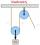On wheels with a diameter of 40 cm is fixed rope with the load. Calculate how far is load lifted when the wheel turns 7 times?
3. Bicycle wheel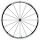Bicycle wheel diameter is 62 cm. How many times turns the bicycle on the road 1 km long?
4. Velocipede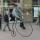The front wheel of velocipede from year 1880 had a diameter 1.8 m. If the front wheel turned again one then rear wheel 6 times. What was the diameter of the rear wheel?
5. Cyclist 9A cyclist travels at a a speed of 4.25 km per hour. At that rate, how far can he travel in 3.75 hours?
6. Knight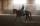Knight passed 13 km long track in 26 minutes, what was his average speed?
7. Pedestrian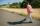Dana started at 10:00 from the point A to point B. These points are distant 12 km. Determine how fast Daniel went when the place B arrived at 11:54. Speed express in km/h.
8. Speed of sound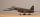The average speed of sound is 330 meters per second. Estimate how long it will hear the church bell 1 km away. Calculate the distance from what would hear sound after 10 seconds.
9. Average speedMichal went out of the house by car at speed 98 km/h. He came into the goal place for 270 minutes. Determine the distance between the two places.
10. CircleWhat is the radius of the circle whose perimeter is 6 cm?
11. Clock handsThe second hand has a length of 1.5 cm. How long does the endpoint of this hand travel in one day?
12. Athlete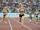How long length run athlete when the track is circular shape of radius 120 meters and an athlete runs five times in the circuit?
13. Circle - simpleThe circumference of a circle is 930 mm. How long in mm is its diameter?
14. Two gears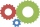The two gears fit together. The larger gear has 32 teeth, the smaller has 20 teeth less. How many times does turn a smaller gear if the bigger gear turns three times?
15. Circle - easy 2The circle has a radius 6 cm. Calculate:
16. Circle from string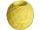Martin has a long 628 mm string . He makes circle from it. Calculate the radius of the circle.
17. Clock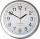How long is trajectory of second hand of hours for day, if is 15 mm long?